# GMAT Math : Calculating the length of the diagonal of a square

## Example Questions

### Example Question #1 : Calculating The Length Of The Diagonal Of A Square

A square has a side length of 3, how long is the diagonal of the square?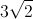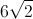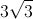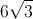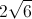Explanation:

The diagonal divides the square into two 45-45-90 triangles, which have side lengths in the ratio of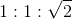. Therefore, the diagonal is the side length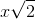.

### Example Question #2 : Calculating The Length Of The Diagonal Of A Square

Given square FGHI below, solve for the following: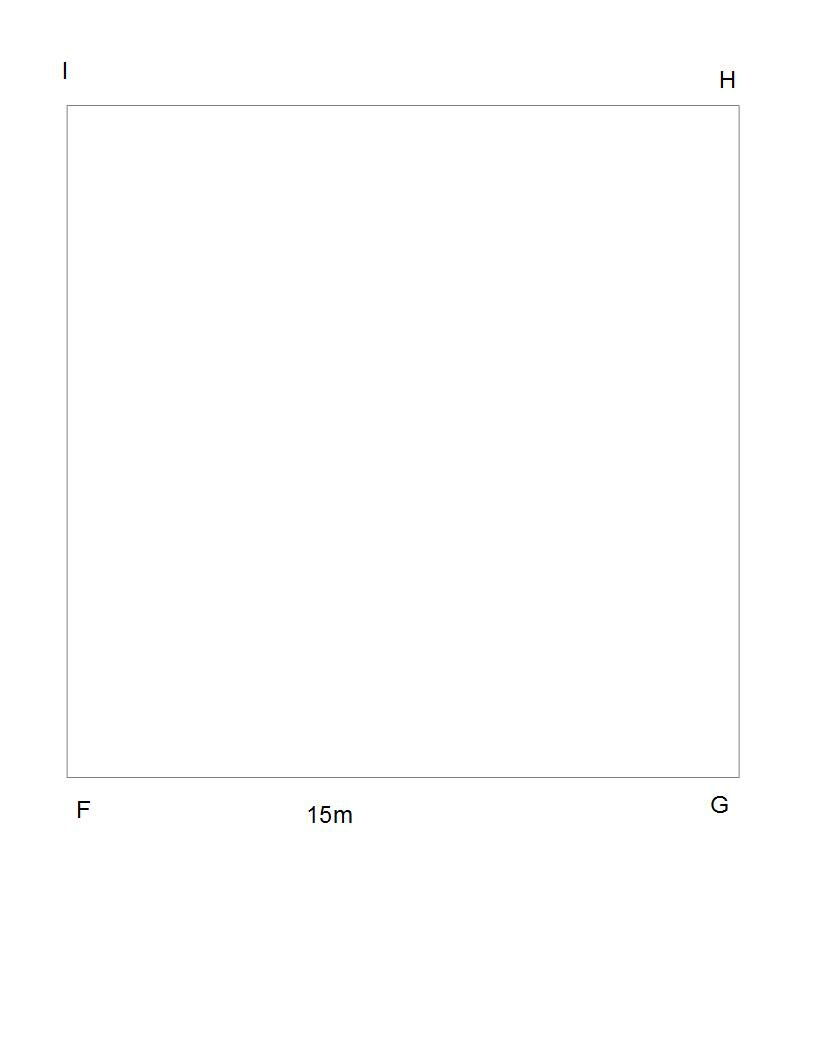If square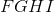represents a grassy area in the middle of your college campus, what is the shortest distance from pointto point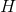?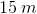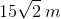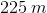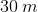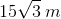Explanation:

There are two pretty easy ways to solve this problem, both of which require you to see that this is actually a triangle question.

The first method is the fastest. If you can recognize that the diagonal of a square makes a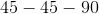triangle, you can then recall thattriangles have side lengths with ratios of. Therefore: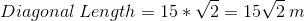The second way to solve this problem is to use Pythagorean Theorem:whereandare leg lengths of a triangle andthe length of the hypotenuse. In this case, we want to solve for the diagonal, which is the hypotenuse of the triangle it forms, so we are solving for: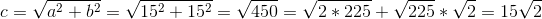So again, we get.

### Example Question #3 : Calculating The Length Of The Diagonal Of A Square

One side of a square has a length of. What is the length of the diagonal of the square?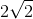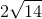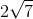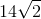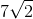Explanation:

By definition, all four sides of a square are the same length. So if one side has a length of, the length and width of the square are both. The diagonal of the square is just the hypotenuse of a right triangle with the length and width as the other two sides, so we can use the Pythagorean Theorem to calculate the length of the diagonal: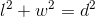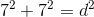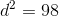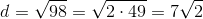### Example Question #4 : Calculating The Length Of The Diagonal Of A Square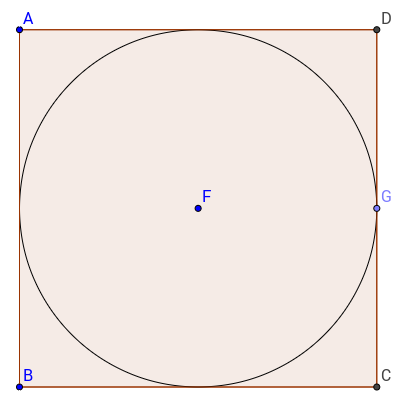A circle is inscribed in a square. The diagonal of the square is, what is the area of the circle?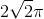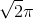Explanation:

From what we are told, we can figure out the side of the square with this simple formula: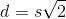, whereis the diagonal of the square andis the length of the side. So the length of the side of our square will be 2. Therefore the radius of the circle will be half that, or 1. Now that we now the radius, we can calculate the area of the circle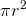, whereis the radius of the circle.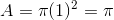### Example Question #5 : Calculating The Length Of The Diagonal Of A Square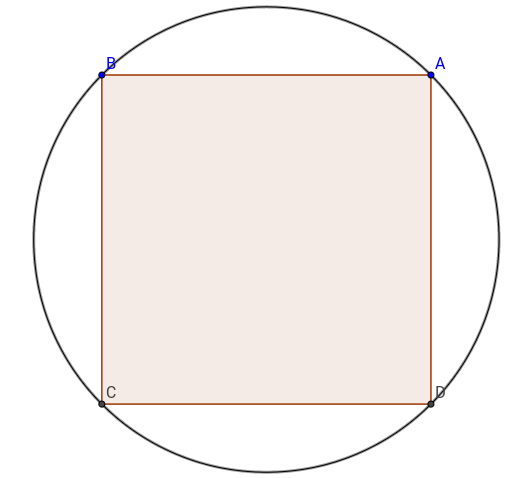A square is inscribed in a circle. The circle has an area of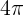, what is the ratio of the area of the square to the area of the circle?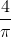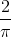Explanation:

To get the area of the square we need to figure out, the length of the side. Which is  possible by calculating the diameter of the circle, which is in fact the diagonal of the square. We can do as follows: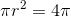thereforethe radius is 2 and the diameter is 4. We now know that the diagonal of the square is 4 as well, with the formula, whereis the length of the diagonal andis the length of the side of the square, we can figure out the length of the side, which is. Therefore, the area is the square of the side or 8. The final ratio that we are looking for is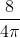or.

### Example Question #6 : Calculating The Length Of The Diagonal Of A SquareA square is inscribed in a circle. The area of the square is. What is the circumference of the semicircle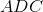?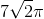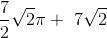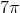Explanation:

From the given area of the square we can figure out the length of the side of this square, which is the square root of the area, or 7. Using the formula for the diagonal of the square,, whereis the length of the diagonal, andthe length of the side. Therefore, the length of the diagonal is. In this case since the square is inscribed in the circle, the diameter of the circle is equal to the diagonal of the square. Therefore, we can find the circumference of the circle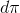, or. We just need to divide the result by 2 since we only want the circumference of the semicircle and also add the length of the diagonal, which "closes" the semicircle. The final answer is.

### Example Question #7 : Calculating The Length Of The Diagonal Of A Square

Find the length of the diagonal of a square whose side length is.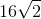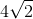To find the length of the diagonal, you can either use the pythagorean theorem or realize that the diagonal of a square is really the hypotenuse of a right isosceles triangle. Therefore, using the short cut, you know that the diagonal is the side length times. Thus, the answer is: